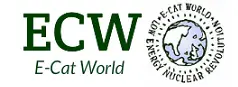# High Current Densities; Environmental Conditions for “Cold Fusion” (Gerhard Hunf)

The following post has been submitted by Gerhard Hunf

High current densities; Environmental conditions for “cold fusion”

The effect of high current densities (J = I / F) on an atom is an e-capture, which leads to the transmutation of the atom. Examples of this are transmutations in conductor explosions and flashes. The cause of the high current density here are extreme currents when compensating extreme potential differences.

However, high current densities can also be achieved by small currents with small potential differences if the current-carrying area is extremely small. A prerequisite for this is, however, a carrier material which can channel this current flow without damage. These are superconductors with Sc> 10exp6A/cm2 and Tc> 293 K.
For practical application, a standard conductor (Cu, Ni, Pd ..) – diameter in the millimeter range – is coated with a superconductor in the nanometer range.

If a current of 1 A flows in the circuit of such a coated conductor, the current branches on the basis of Kirchhoff’s law:I1/I2=R2/R1

Assume (R1=10exp-2Ωmm2/m; R2=10exp-20Ωmm2/m), than you get:

10exp-18 A to the standard line and 0.99999 A on the superconductor line..

This means, the current flows almost completely through the nm cross-section of the superconductor.

To determine the area of the nm cross-section, calculate the area of the circular ring that the superconductor forms around the normal axis:

FKreisring = π(R2 – r2)
R2-r2 = 0.000000000001 m2 = 10exp-11 m2

The current density in the superconducting layer is thus:

J=1A/10exp-11 m2=10exp11 A/m2

The coating of the substrates with the necessary superconductors can performed as described in DE102008047334B4.